[BACK]
 Intelligent Automation & Soft ComputingDOI:10.32604/iasc.2023.025618Article

Minimizing Buoyancy Factor of Metallic Pressure-Hull Subjected to Hydrostatic Pressure

1Department of Mechanical Engineering, Faculty of Engineering, Taif University, Taif 21944, Saudi Arabia
2Production and Mechanical Design Department, Faculty of Engineering, Mansoura University, Mansoura 35516, Egypt
3Civil Engineering Department, M.T.C. Kobry Elkobba, Cairo 11865, Egypt
4Ships and Submarines Engineering Department, Military Technical College, Cairo 11865, Egypt
5Civil Engineering Department, Sohag University, Sohag 82524, Egypt
6Department of Civil and Environmental Engineering, Incheon National University, Incheon 22012, Korea
7Incheon Disaster Prevention Research Center, Incheon National University, Incheon 22012, Korea
8Mechanical Engineering Department, Faculty of Engineering & Technology, Future University in Egypt, Cairo 11835, Egypt
*Corresponding Author: Jong Wan Hu. Email: jongp24@inu.ac.kr
Received: 30 November 2021; Accepted: 17 February 2022

Abstract: To increase the payload, reduce energy consumption, improve work efficiency and therefore must accordingly reduce the total hull weight of the submersible. This paper introduces a design optimization process for the pressure-hull of submarines under uniform external hydrostatic pressure using both finite element analysis (FEA) and optimization tools. A comprehensive study about the optimum design of the pressure hull, to minimize the weight and increase the volume, to reach minimum buoyancy factor and maximum operating depth minimizing the buoyancy factor (B.F) is taken as an objective function with constraints of plate and frame yielding, general instability and deflection. The optimization process contains many design variables such as pressure-hull plate thickness, unsupported spacing, dimensions of long and ring beams and finally the elliptical submersible pressure-hull diameters. The optimization process was conducted using ANSYS parametric design language (APDL) and ISIGHT. The Multi-Island Genetic Algorithm (G.A) is considered to conduct the optimization process. Additionally, parametric analysis is done on the pressure hull to examine the effect of different design variables on the pressure-hull design. As a result, the B.F of the proposed optimal model is reduced by an average of 31.78% compared with Reference Model (RM). Maximum von Mises stress is reduced by 27% as well. These results can be helpful for submarine pressure-hull designers.

Keywords: Buoyancy factor; pressure-hull; material failure; ANSYS; ISIGHT; general instability

1  Introduction

The weight of the hull is an essential factor of the submarines. It enables the submarines to withstand hydrostatic pressure. The hull weight should be about half or less the total weight of submarines in dry condition. Therefore, it is vital to increase the efficiency of the structure to enable more loads carried in submarines. Working at high operating depths requires the perception of how hydrostatic pressure can be affected on both the structural and materials. Submarines experience varying hydrostatic loads while in operation due to diving therefore the pressure hull must be designed to achieve safety, reserve buoyancy and space efficiency [1,2]. The diving depth is one of the most important criteria for designing the submarine pressure-hull . One of the main difficulties confronted by the designer of deep-submersibles is to decrease the weight and increase the payload. Minimum structural weight is preferred by the designer, those are same with the minimum buoyancy factor [4,5]. The machinery contributes approximately only one-third of the total dry weight. The pressure-hull is the major structural element of the submerged vehicles and forms approximately more than one-half of the entirety weight . Therefore, the minimum B.F design for the pressure hull aims to make the spaces that use and operate the submarines, more efficient. Therefore, the velocity and the payload can be increased . The payload is considered as accommodation, weapon stowage and launching arrangements. The designer of submerged vehicles illustrated that the safety factors ranging from 1.50–2.00 were considered acceptable in most engineering practices . Elsayed et al. [8,9] worked on optimization of stiffened pressure hulls made up from composites and used the Sub Problem Approximation (SPA) technique to reduce the buoyancy factor. Helal et al.  optimized a sandwich composite pressure hull to minimize the B.F and maximize the buckling strength factor and deck area. It is noted that at extreme depths the core thickness plays a minor role in the design of composite deep pressure hull. Helal et al.  presented a methodology for the multi-objective optimization of cross elliptical pressure hull to maximize buckling capacity and minimize the B.F. Several earlier researches have focused on solving and predicting different problems and models as in . Moreover, Helal et al.  presented the optimization procedure for increasing the buckling capacity, and minimizing the (weight and drag force). Li et al.  performed a collaborative optimization to minimize the composite pressure hull weigh. Jung et al.  analyzed and presented a method for buckling pressure calculation of a filament wound cylindrical shell subjected to hydrostatic pressure. Liang et al.  presented the optimization design of a composite cylindrical shell under hydrostatic pressure. Imran et al. [21,22] conducted the optimization of a composite pressure-hull for increasing the buckling load factor and reducing the Buoyancy Factor (B.F). Moreover, Helal et al. [10,11,23] conducted the optimization analysis on a stiffened composite pressure-hull to minimize B.F. The material failure and buckling load factor were employed as constraints in the optimization process. Additionally, Garland  demonstrated that not only the weight to buoyancy ratio plays an important role, but also a higher strength material requires less weight. Furthermore, Lee et al.  optimized a composite cylinder under external hydrostatic pressure.

Do et al.  derived an empirical equations to predict the residual ultimate strength of both stringer and ring stiffened cylinder under hydrostatic pressure, moreover found that the stringer-stiffened cylinder was failed due to the local buckling at the stringer stiffener panels, whereas the ring-stiffened cylinder was failed due to the overall buckling mode. Wei et al.  optimized the cross-sectional shape of the stiffeners of a composite pressure hull to improve the stability and maximize the buckling pressure and found that the stability was improved when the bottom angle of the trapezoidal stiffener is nearly one hundred degrees. Muttaqie et al.  studied the optimum design of a steel pressure hull to find the optimum weight and buoyancy coefficient based on the interactive nonlinear collapse strength analyses. They used different materials at different operational design depths. It is found that the materials with higher strengths show smaller buoyancy coefficients as depths increase. Muttaqie et al.  investigated the failure behavior of ring-stiffened cylinder subjected to hydrostatic pressure. The local buckling pressure, overall buckling pressure, and yield pressure were examined. Viljoen et al.  investigated the effect of corrosion thinning on the collapse pressure hull made of steel and predicted that the submarine hull would buckle at a depth of 567 m when the nominal plate thickness is 25.4 mm, Owing to corrosion thinning, the collapse pressure was reduced by 27 kPa per annum. Bagheri et al.  applied the genetic algorithm (G.A) method to a multi-objective optimization problem of ring stiffened cylindrical shells. Walker et al.  described multi-objective techniques for combining both G.A and Finite Element Method (FEM) to minimize the weight and deflection. Costa et al.  described the application of a genetic algorithm (G.A) to material and sizing optimization. Messager et al.  studied the design optimization of the submarine to maximize the buckling pressure by using “G.A”. Jen  studied the optimization of a pressure-hull with a ring-stiffened cylinder subjected to hydrostatic pressure. Adali et al.  conducted an approach to optimize a laminate cylindrical pressure-hull. Moon et al.  studied the buckling behavior of a composite cylinder subjected to hydrostatic pressure. Zhang et al.  examined the buckling behaviours of six segmented toroids and a continuous toroid under external pressure. The results showed a good agreement between the experimental observations and the numerical estimations. Maalawi  showed a mathematical model for enhancing the buckling stability of composite cylinders under external pressure to maximize the critical buckling pressure. Lee et al.  incorporated the optimization to increase the design load of composite cylinders taking into consideration the material failure and buckling. The optimization of a composite cylindrical pressure-hull was studied to minimize the weight of pressure-hull structure through utilizing the multi-island genetic algorithm technique . On the other hand, a reduction in the overall collapse and yield pressure of about 20% and 40%, respectively, was found as a conclusion of studying the effect of corrosion on the stability and strength of pressure-hulls . Panteleev  introduced an optimization for sandwich structures and assured a reduction by 23% in its weight by 23% compared to the constant thickness plate. Besides, a higher strength material and stiffness was found to provide better buoyancy and less weight . Geuskens et al.  showed the interior configuration of the multi-bubble pressure cabin in detail, and analyzed it under internal pressure. The multi-bubble is composed of cylindrical, spherical, toroidal and tapered membrane elements.

From previous literature, B.F has a significant effect to enhance space utilization of submarines under hydrostatic pressure and optimizing submerged pressure-hull. It is believed that BF needs more investigations. In present work, a new optimal numerical model is established to minimize B.F of a metallic deep-submerged pressure-hull subjected to hydrostatic pressure. This study presents some design suggestions based on the results of design variables effects on each different physical quantity. Some design variables show little influence. So that, they can be omitted to simplify the objective function and accelerate the optimization process. Furthermore, all results are helpful in future design and development of submersible pressure-hull under uniform external hydrostatic pressure. The remainder of this paper is arranged as follows. In Section 2, some important design basics of submerged pressure-hull are introduced. The failure mechanism is defined in Section 3. Optimization procedures are presented in Section 4. In Section 5, Finite Element modeling and simulation for the proposed model is conducted using ANSYS to obtain a Reference Model (RM). Then, an optimization study is developed using both ANSYS and ISIGHT, considering the Multi-Island Genetic Algorithm. The results and conclusions are discussed in details in Section 6.

2  Shapes and Material Requirements for Submarine Pressure-Hull

The forms of pressure hull structure, including spherical hull structure, ellipsoidal hull structure, cylindrical hull structure, toroidal hull structure and composite hull structure are shown in Fig. 1 . Cylindrical shapes are usually used for submarine pressure hulls rather than spheres as they provide a good compromise between the structural efficiency and the internal space. The hull structural weight is one such parameter in that decreasing weight reduces the power demand throughout the service life . Several pressure-hull arrangements that can be used in submersible bodies are illustrated in Fig. 2 . The ring-stiffened hulls have better structural performances and are commonly used in submarines and underwater vehicles [49,50]. The structural outline of a submarine pressure-hull is in the form of a ring-stiffened circular cylinder, and blocked by end caps as illustrated in Fig. 3 .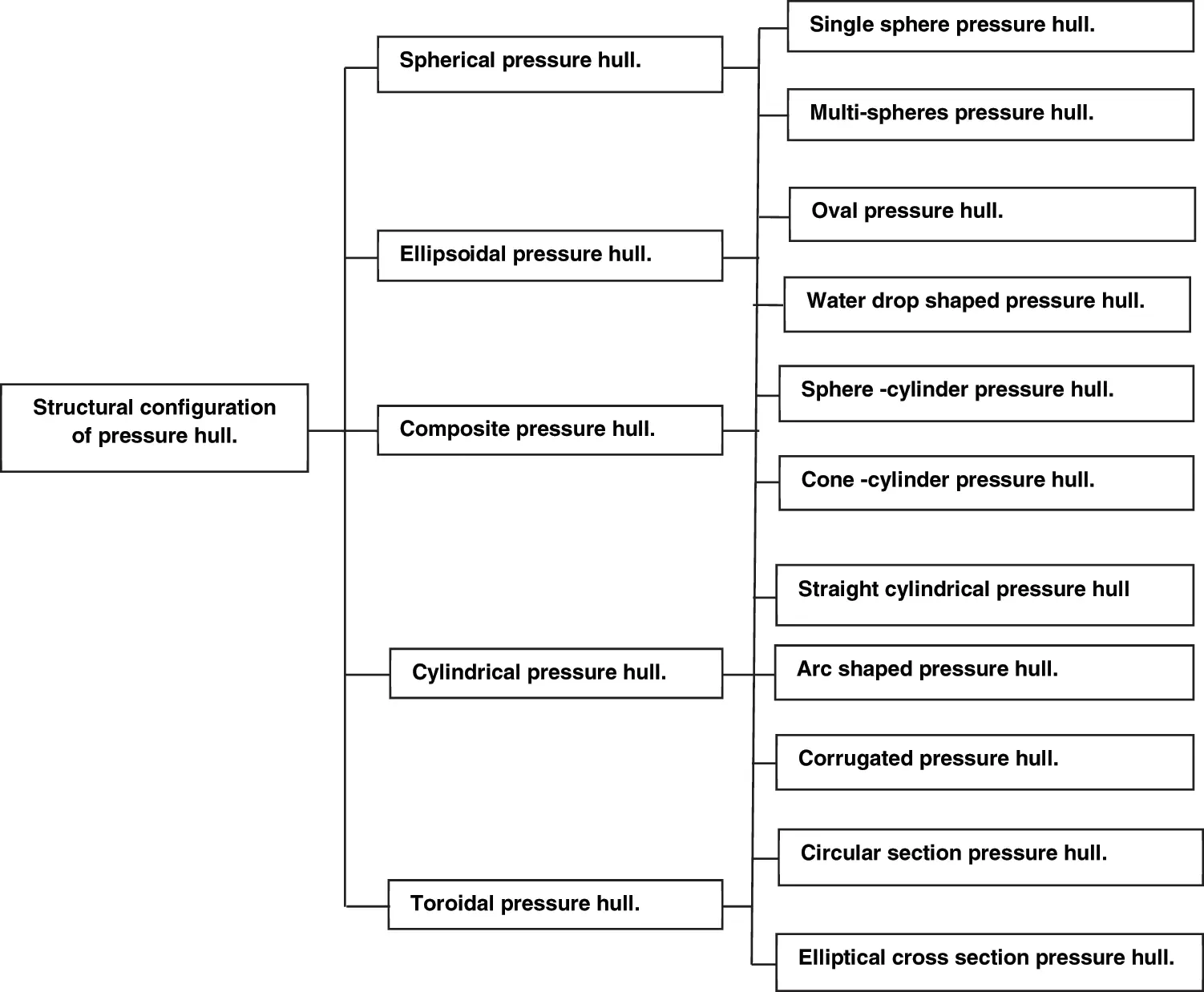Figure 1: Structural classification of pressure hulls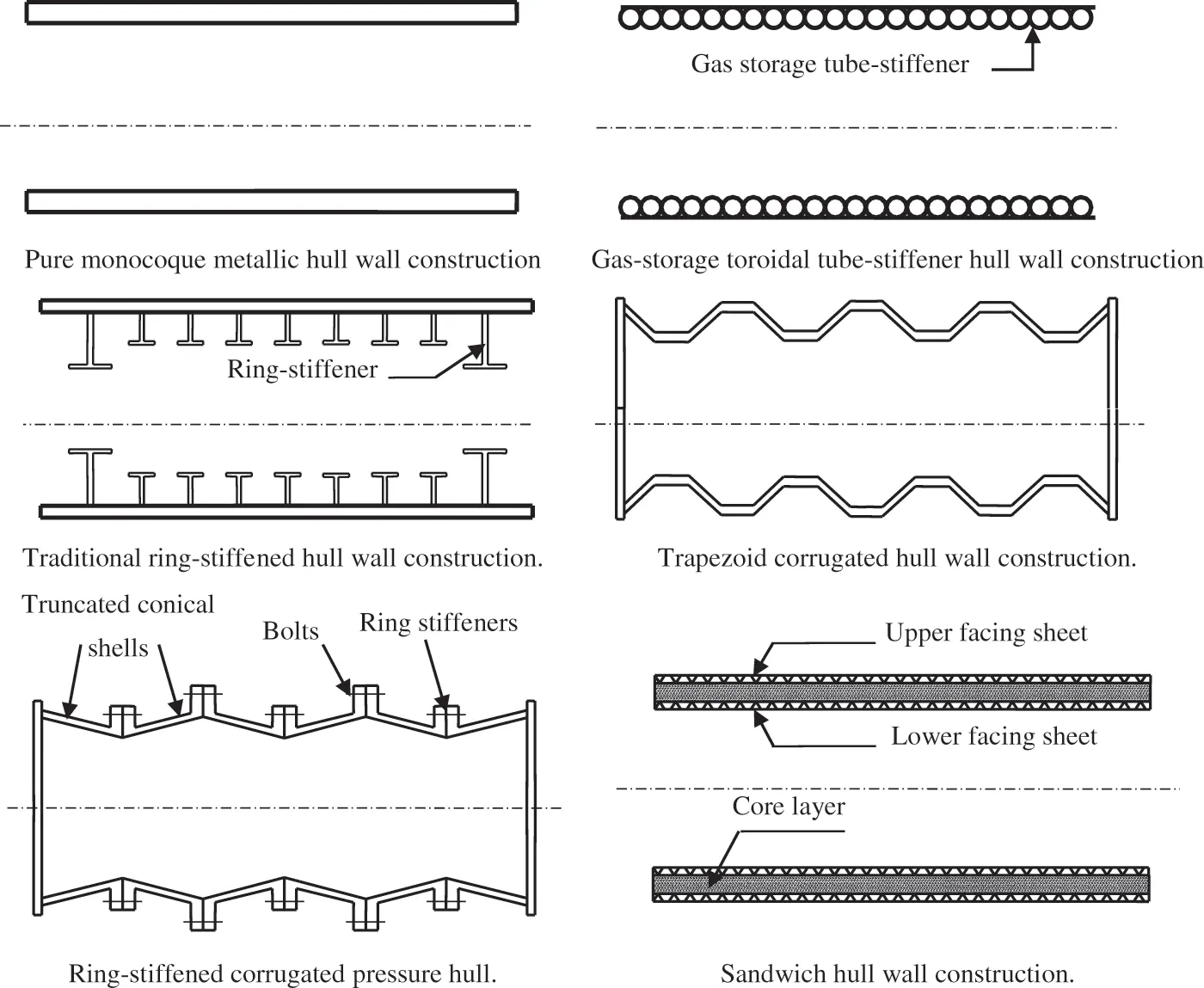Figure 2: Shape of various wall architectures used for pressure hullsFigure 3: Usual shape of a submarine pressure hull

In this paper, a pressure-hull in the form of the elliptical cylinder is selected as demonstrated in Fig. 4. The materials used in manufacturing the underwater pressure-hulls must be capable of withstanding the external pressures, and also have a proper property to withstand the surroundings . If the submarines and underwater vehicle’s wall thickness is large, the submarines and underwater vehicles will be sinking like stone. The main materials for submarine pressure-hulls are composites, high strength steels, titanium alloys and aluminum alloys .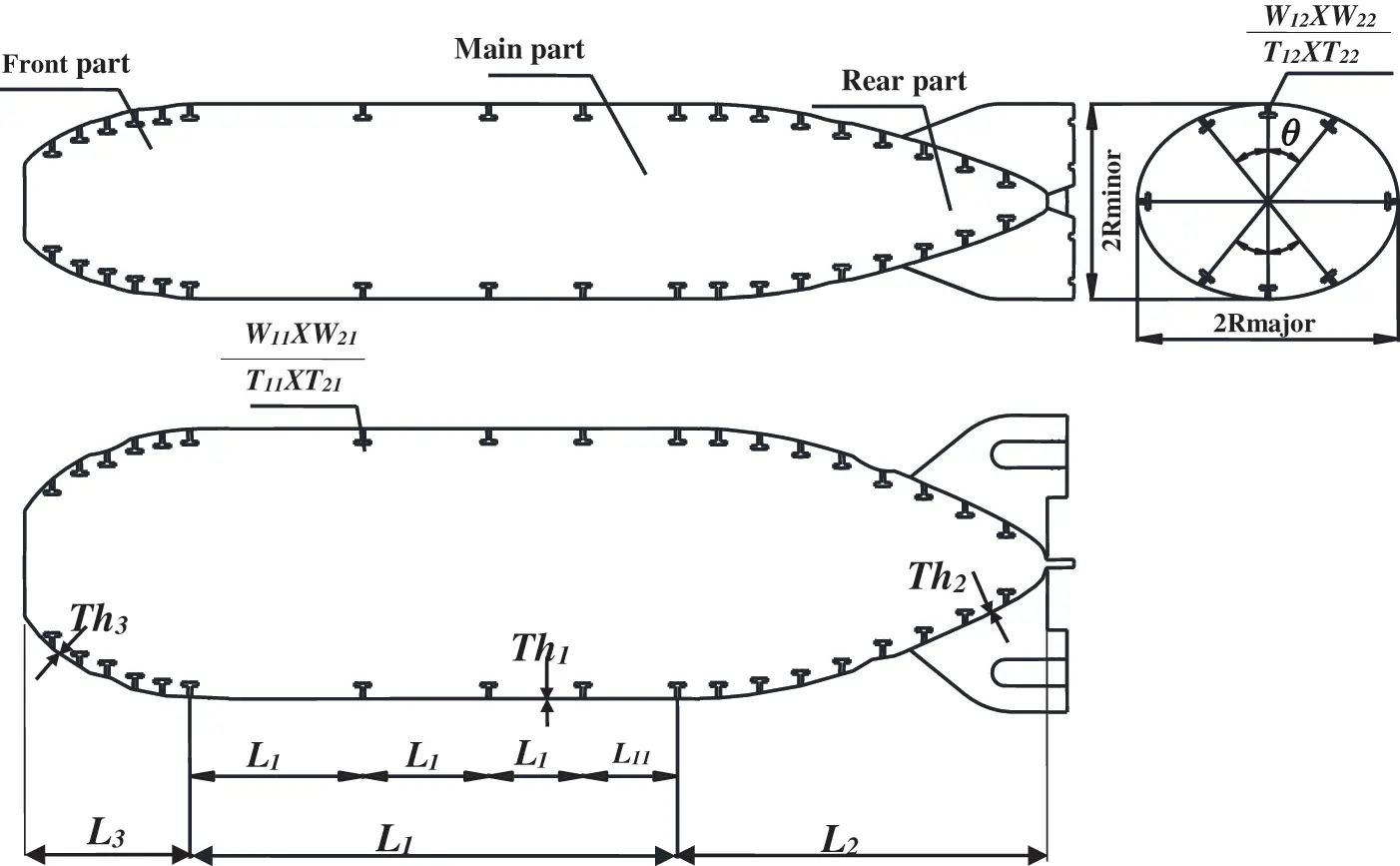Figure 4: Geometry of the pressure-hulls

The relationship between the collapse depth and B.F for an un-stiffened cylinder constructed from different materials (HY80, GRP and CFRP), in case of both material and buckling failures, is shown in Fig. 5 . In the present study, the model was constructed from HY100 for the hull shell, and titanium alloys for the stiffeners.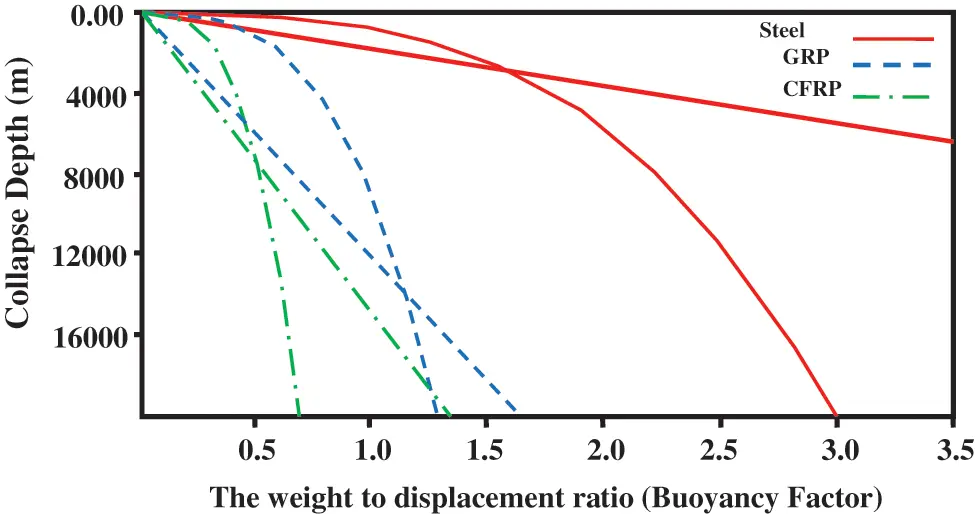Figure 5: Weight to displacement ratio vs. collapse depth for un-stiffened cylinders

The Titanium alloy has high strength, good corrosion resistance and light weight compared with HY100. The stress-strain curves for HY100 and titanium alloys are illustrated in Figs. 6a, 6b [53,54]. Furthermore, the properties of the materials are presented in Tab. 1 [55,56].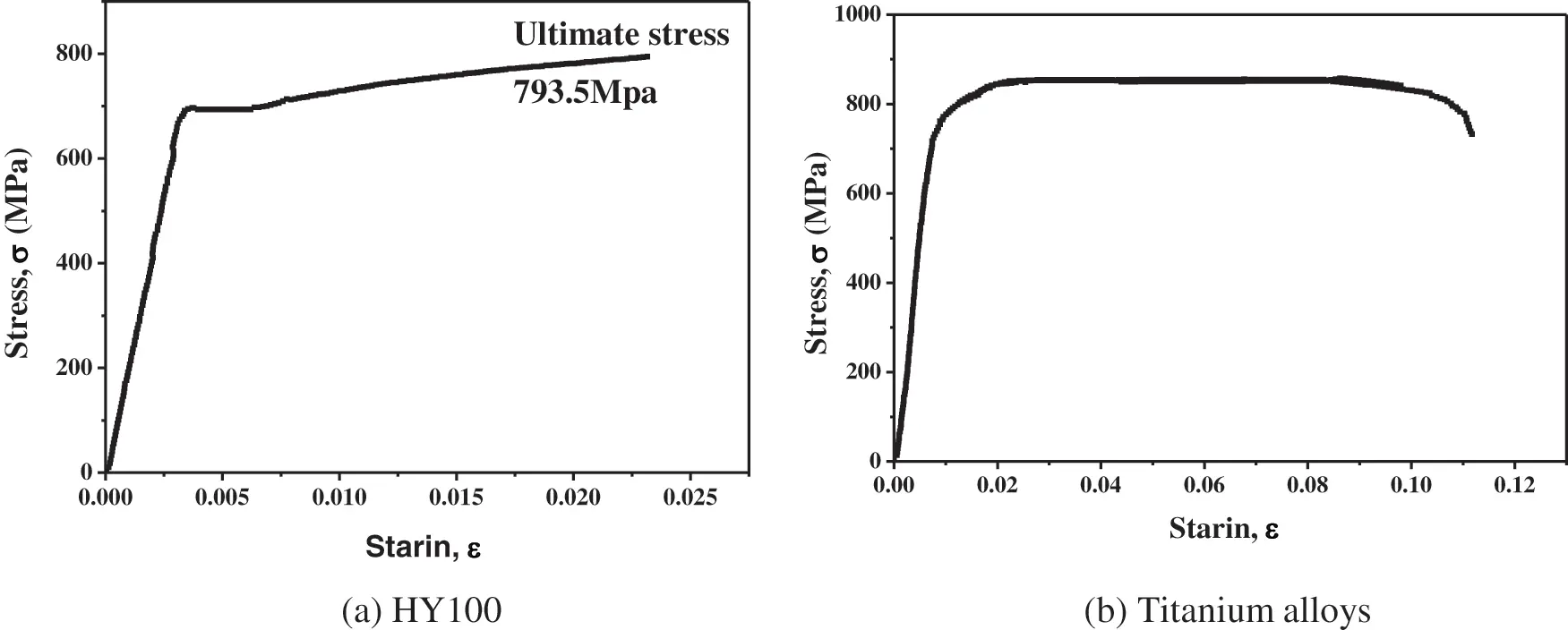Figure 6: The stress strain curve for both HY100 and Titanium alloy3  Failure Mechanisms

The failure modes which governs the design procedures of the pressure-hull is related with a sudden loss of stability, wherever the pressure-hull can no longer hold its shape and suddenly collapses to a shape with less internal volume, under a constant load. This failure is defined as buckling. To enhance the buckling strength of the pressure-hull shells is to stiffen with ring stiffeners . The failure mode of particular ring-shell geometry will depend on the shell-thickness-to-radius ratio, the ring beam-spacing-to-the shell-radius ratio, the ring cross-sectional-area to the shell cross-sectional area ratio additionally, the geometric, the materials properties and material imperfections . The first failure mode is an accordion-type pleat around the circumference between adjacent rings, and it can occur in more than one bay. The 2nd failure mode is lobar buckling of the pressure-hull which is a phenomenon associated with a sudden loss of stability across the shell, which can occur for a lower pressure than what it would require the vessel to yield and happens between the adjacent rings, resulting in the appearance of lobes around the circumference of the shell . A further source of pressure hull instability is frame tripping, which refers to the torsional buckling of an inadequately proportioned ring-stiffener. The 3rd failure mode is the general instability, in which both the shell and the ring beams can fail if the ring beams are not strong enough. This is defined as critical buckling pressure; Pcr. Fig. 7 shows the typical pressure hull structure and buckling modes . For an infinitely long circular cylinder under uniform radial pressure, the critical buckling pressure is given as in  and defined as follow:

Pcr=E4(1ν2)(tRm)3 (1)

where: Pcr denotes the critical buckling pressure in N/mm², t denotes the shell thickness of the cylinder cross-section in mm, Rm denote the mean radius of the shell in mm, E denotes Young’s modulus in N/mm² and ν denotes the Poisson’s ratio. The following Greiner analytical Eq. (2) given in  is the most accurate instability formula for a cylindrical shell under hydrostatic pressure.

Pcr=E(tR)n2(1(1+(n2π2)(1r)2)2+(tR)212(1ν2)(n+π2(rl)2)2) (2)

where: r, denotes the radius of the pressure-hull shell in mm, l, denotes the length of the pressure-hull shell in mm and n, denotes the number of circumferential lobes.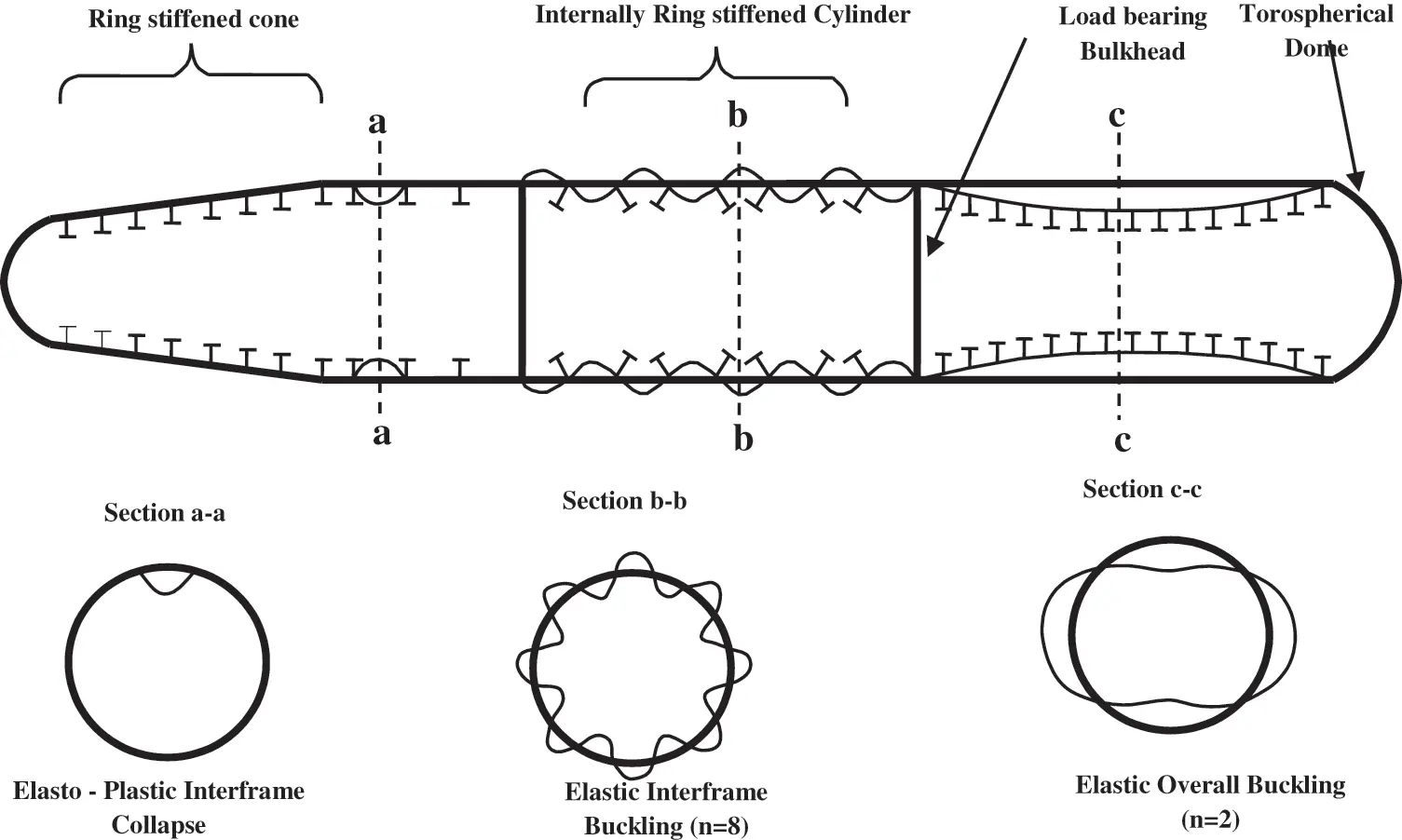Figure 7: Typical pressure hull structure and buckling modes

For predicting the general instability failure mode Eqs. (3) and (4) were used namely Bryant and Kendrick Equations as follow.

a)   Bryant Equation .

Pcr=EtRπ4R4L4(n2+π2R22L21)(n2+π2R2L2)2+EIe(n21)LN+1(R+t2)(R+ec)2 (3)

b)   Kendrick Equation 

Pcr=EtRπ4R4L4(n2+π2R22L21)(n2+π2R2L2)2+EIe(n21)LN+1R3 (4)

where: (Ie) denotes the effective moment of inertia comprising one stiffener plus an effective shell width in mm4. L, denotes the cylindrical shell Length in mm. R, denotes the cylindrical shell radius in mm. N, denote the number of the ring beams. ec, denotes the stiffeners and the shell effective width eccentricity. To ensure stability, the critical buckling strength (Pcr) must be exceeding the actual load (Pact) and the calculated critical pressure multiplied by (0.75) when used in the design .

One of the yield criteria should be used to predict the actual structural failure, which defines the allowable state of stress for a material. For isotropic structures, von Mises yield criterion is the most commonly used criteria based on the distortion energy theory. Therefore, von Mises yielding criteria is used in this work to evaluate the capability of the pressure-hull to resist the yielding failure. The failure index (IF) is calculated as following :

IF=σ112σ11σ22+σ222σ0 (5)

where:σ0 is the material yielding strength, σ11 and σ22 are the principal stresses. In this study, both von Mises and buckling load multiplier (λ=PPcr) are incorporated to verify the static failure.

4  Design Optimization Procedures

The optimization method introduces optimal design from all available possible designs . Like other genetic algorithms, MIGA represents each design point as an individual with a certain value of fitness, based on the value of an objective function and constraint penalty. The design optimization problem of the pressure-hull is to minimize the whole weight of submersible pressure-hull under constraints of failure and buckling strength. Fig. 8 shows the optimization flow chart for the elliptical pressure-hull. The structural model was run with four different mesh sizes element, representing 300, 200, 100 and 50 mm to check the convergence. It is seen that variation in displacement and stresses values are insignificant, therefore simulation was carried out with 100 mm average element size.Figure 8: Design optimization flow chart

4.1 Design Variables

Design variables are parameters that can change the specifications of the model within a pre-defined range during the optimization process. In the present study, the design variables are the distance between ring stiffener (Li) of the cross-elliptical pressure-hull, the radii of the ellipse of the elliptical submersible pressure-hull (the maximum diameter “Rmajor” and the minimum diameter “Rminor”), the ring and long beam dimensions (W11, T11, W21, T21, W12, T12, W22 and T22), the stiffeners orientation angle (θ) and the shell thickness of each part of the submersible pressure-hull (Th1, Th2 and Th3).

4.2 Objective Function

The objective function F(x) of the optimization used in this study is to minimize the buoyancy factor (B.F). The buoyancy factor has been utilized as an optimization objective in recent research . If the weight-to-displacement ratio (B.F) is equal to or smaller than unity, the structure will float; otherwise, it sinks like a stone. The buoyancy factor is calculated by dividing the weight of the pressure-hull by the weight of the water displaced by the pressure-hull as shown in Eq. (6).

F(X):Minimize[TotalpressurehullweightTheweightofthefluiddisplacedbythevolumeofthepressurehull] (6)

4.3 Design Constraints

Generally, the requirement that need to be satisfied in the design is the safety of the hull structure under loading conditions. In this study, the constraining equations in the optimization can be classified into three types of constraints, namely: material strength, instability and side constraints. To prevent failure of material for shell and stiffeners, von-Mises stress (σv) must be smaller than the yield stress (σy) of the steel and titanium alloy. The material constraints are denoted by Eq. (7).

σy σv (7)

The buckling load multiplier (λ) is considered as a structural instability constraint and must be equal to or higher than 1. Therefore, a general instability of elliptical submersible pressure-hull is presented as:

λ=PPcr1 (8)

where: (Pcr) is the minimum critical buckling load and (P) is the actual operating loading. For side constraints the higher and lower limits of different variables are shown in Tab. 2.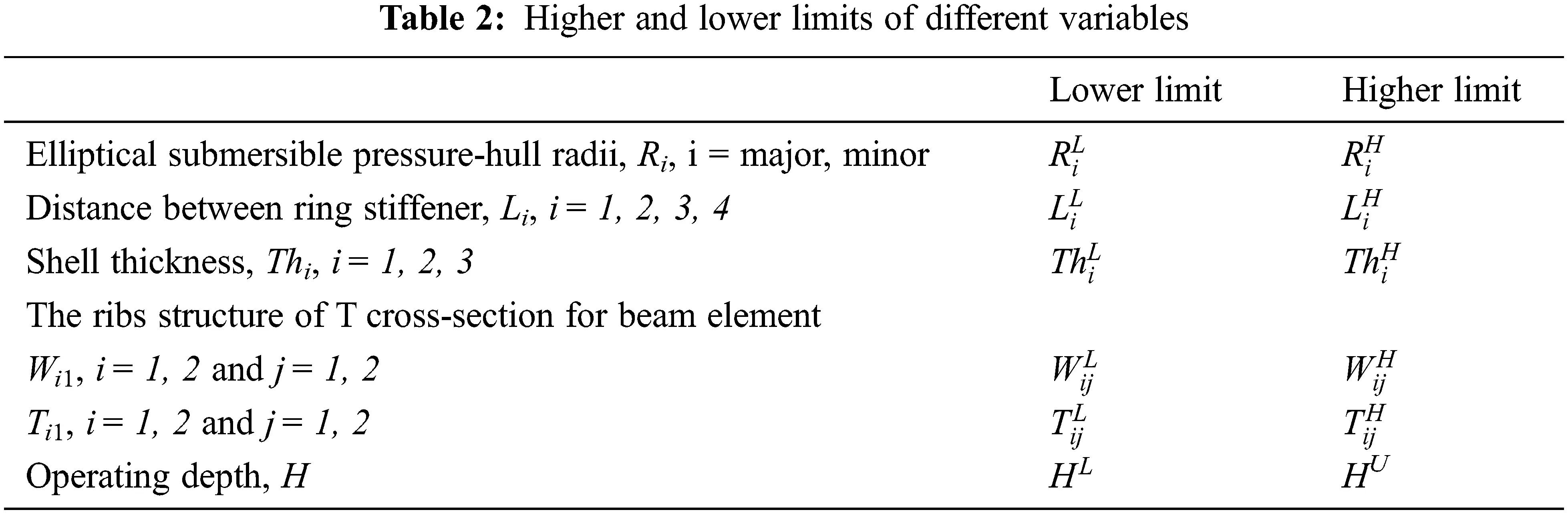5  Finite Element Modeling of the Pressure-Hull and Numerical Simulation

The FEM is generally used for solving numerous problems of engineering furthermore, predicting the behavior of the structural elements . As illustrates in Fig. 9, the FEM of the pressure-hull is constructed in ANSYS. The pressure-hull was modeled using BEAM189 for stiffeners and SHELL93 for the shell [72,73]. Beam and shell mesh for the full model are illustrated in Fig. 10. It consists of 20828 elements and 55840 nodes. The average element size employed in the ANSYS was 0.1 m. The boundary conditions applied within the model were a symmetry boundary condition in all nodes at “X-Z plane” and “Y-Z plane”. Moreover, due to the fluid flow in the “Z-direction”, one single node was constrained in Z-direction according to studies [10,74].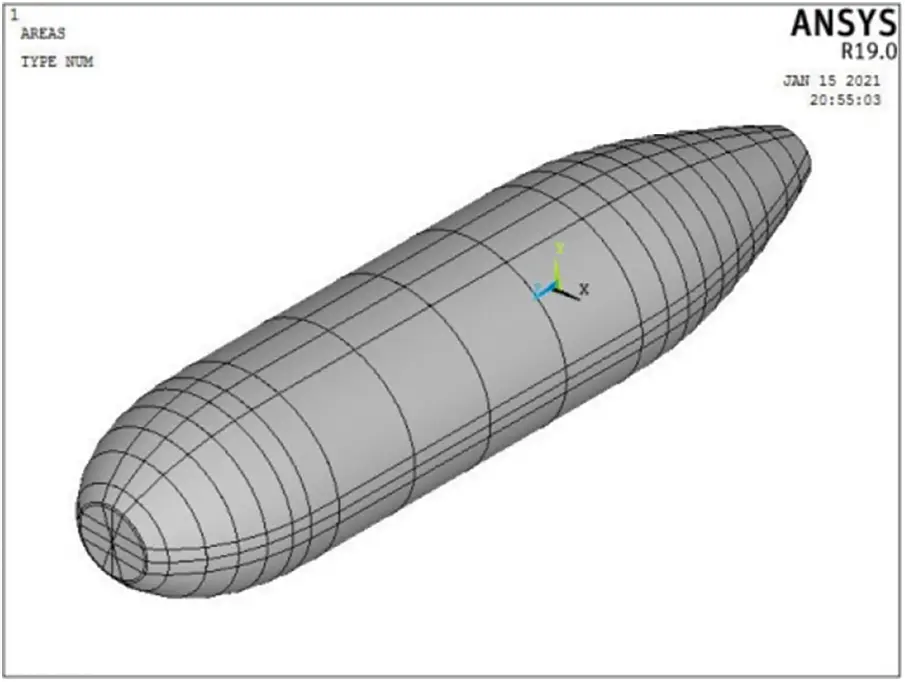Figure 9: The finite element model of the hull structure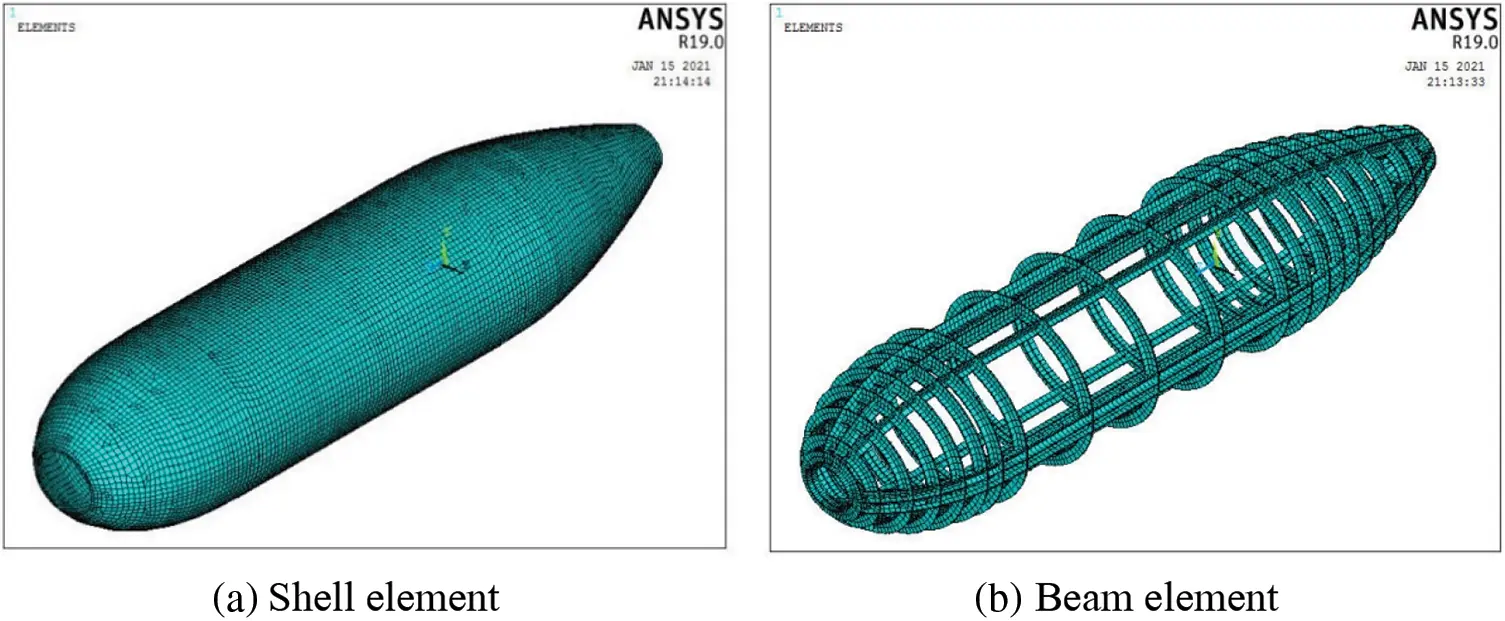Figure 10: The mesh of the global model

6  Results and Discussion

Results of the RM using ANSYS are illustrated in Tab. 3. The optimal objective function (buoyancy factor (B.F)) is 0.601. Results of optimal design model using ANSYS and ISIGHT are illustrated in Tab. 4. Results revealed that the optimal objective function (B.F) is equal to 0.41. Furthermore, Results can overcome all structural failures until the operating depth of 1772.5 m. Furthermore, the table indicates that the buckling strength factor is equal to 5.35, and the maximum permissible deflection is equal to 6.2 mm. In addition, the major diameter Rmajor is equal to 1600 mm, and the minor diameter Rminor is equal to 1400 mm. The findings from these results revealed that the B.F is reduced by 31.78%. Additionally, maximum von Mises stress is reduced by 27%. Fig. 11 presented the output responses of the maximum deflection due to design variables during the optimization processes. For operating depth (H), the distance between ring stiffeners L1 and major diameter, the maximum deflection value increases as the aforementioned parameters increased and vice versa for minor diameter (Rminor), stiffener height (T11) and Shell thickness (Th1). Additionally, a sensitivity analysis is performed to thoroughly the results of the planning variables upon the structural strength of the pressure-hull.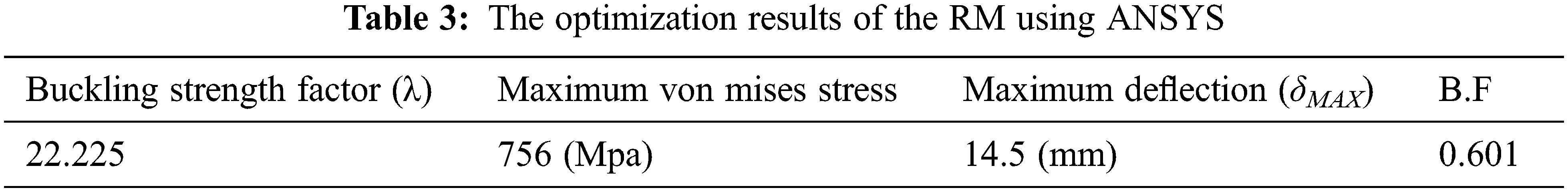Figure 11: Output responses of maximum deflection due to design variables during optimization processes

Deformation distribution over the global model is presented in Fig. 12. It reveals that displacement distribution in X-direction over the global model is approximately equal to displacement distribution in Y-direction with maximum values 5 and 6 mm, respectively. Furthermore, the maximum magnitude of the displacement occurs at the centroids regions of the submarine’s hull. Additionally, displacement distribution in Z-direction for the global model is too small and equals to 0.88 mm. The deformation distribution over the ring and long beams in X, Y and Z direction were presented in Fig. 13 and reveals that the maximum magnitude of the displacement occurs at the centroids regions for both X and Y directions. Additionally, the displacement distribution in Z-direction equals to 0.88 mm. Furthermore, Fig. 14 presents the principal stresses and von Mises stresses distribution. The maximum value in case of the first principal stress distribution is larger than the maximum value of the second principal stress distribution. Additionally, the third principal stress distribution over the global model is nearly uniform distribution.Figure 12: Deformation distribution over the global model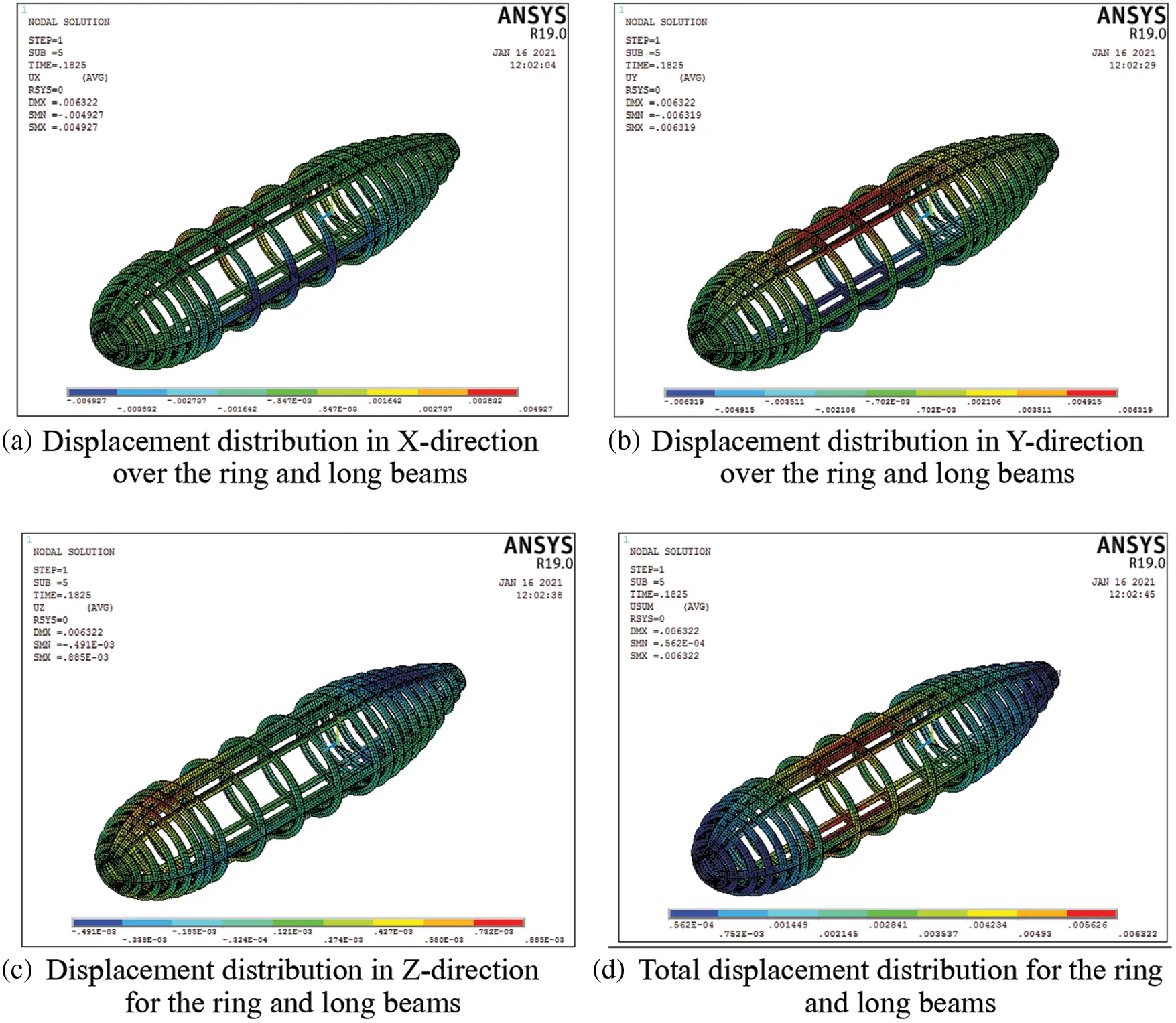Figure 13: Deformation distribution over the ring and long beams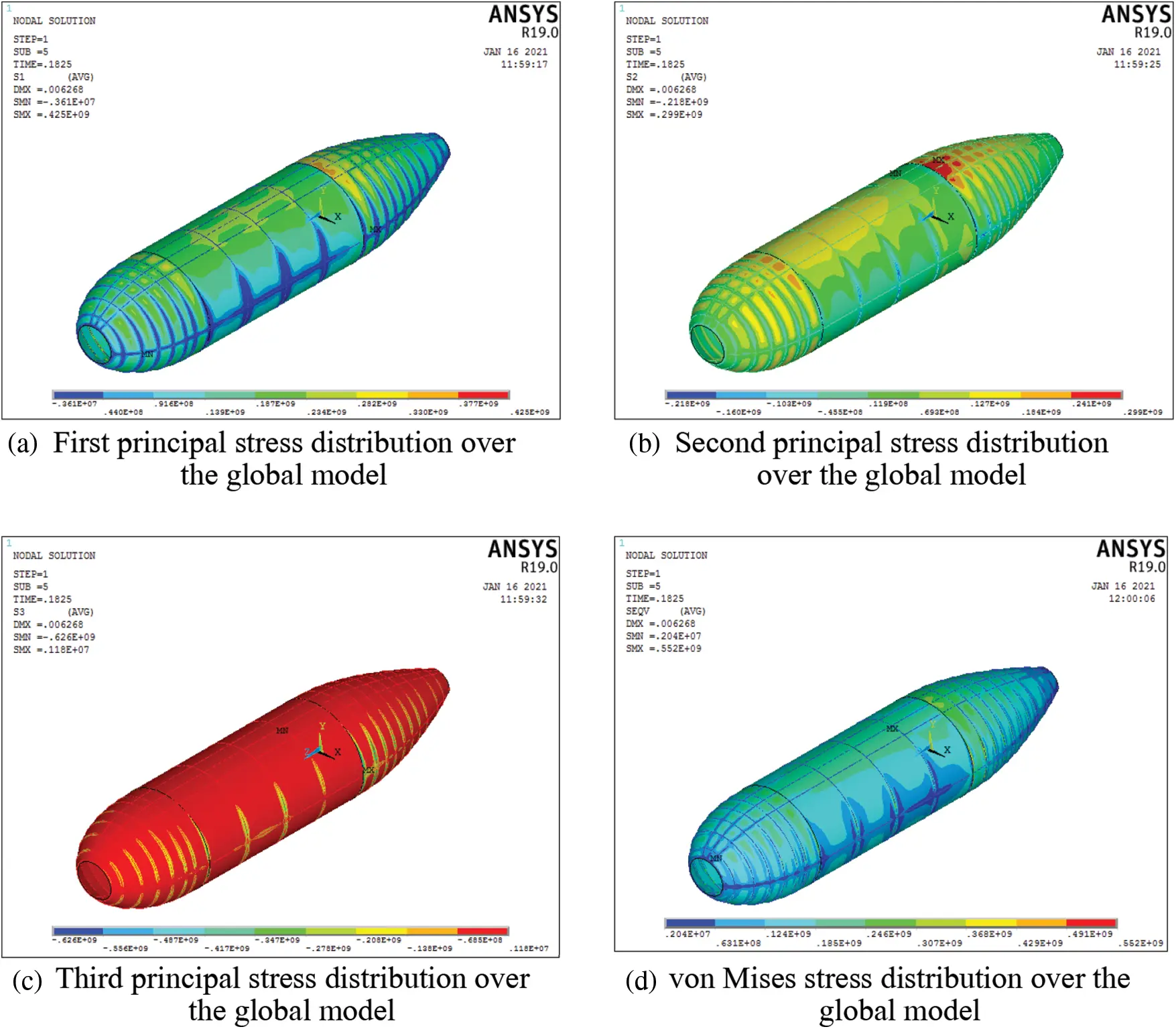Figure 14: Stress distribution over the global model

6.1 The Effect of Design Variables on B.F

The B.F is describing the efficiency of the submarine pressure-hull. The critical value of B.F is one. Fig. 15 demonstrates that B.F is directly proportional to shell thickness (Th1) and ring beam width (W11 and W21). Additionally, B.F is inversely proportional to the major diameter (Rmajor) and minor diameter (Rminor). A larger shell thickness (Th1) or ring beam width (W11 and W21) implies a higher B.F. Furthermore, increasing the major diameter (Rmajor) and minor diameter (Rminor) can decrease the B.F.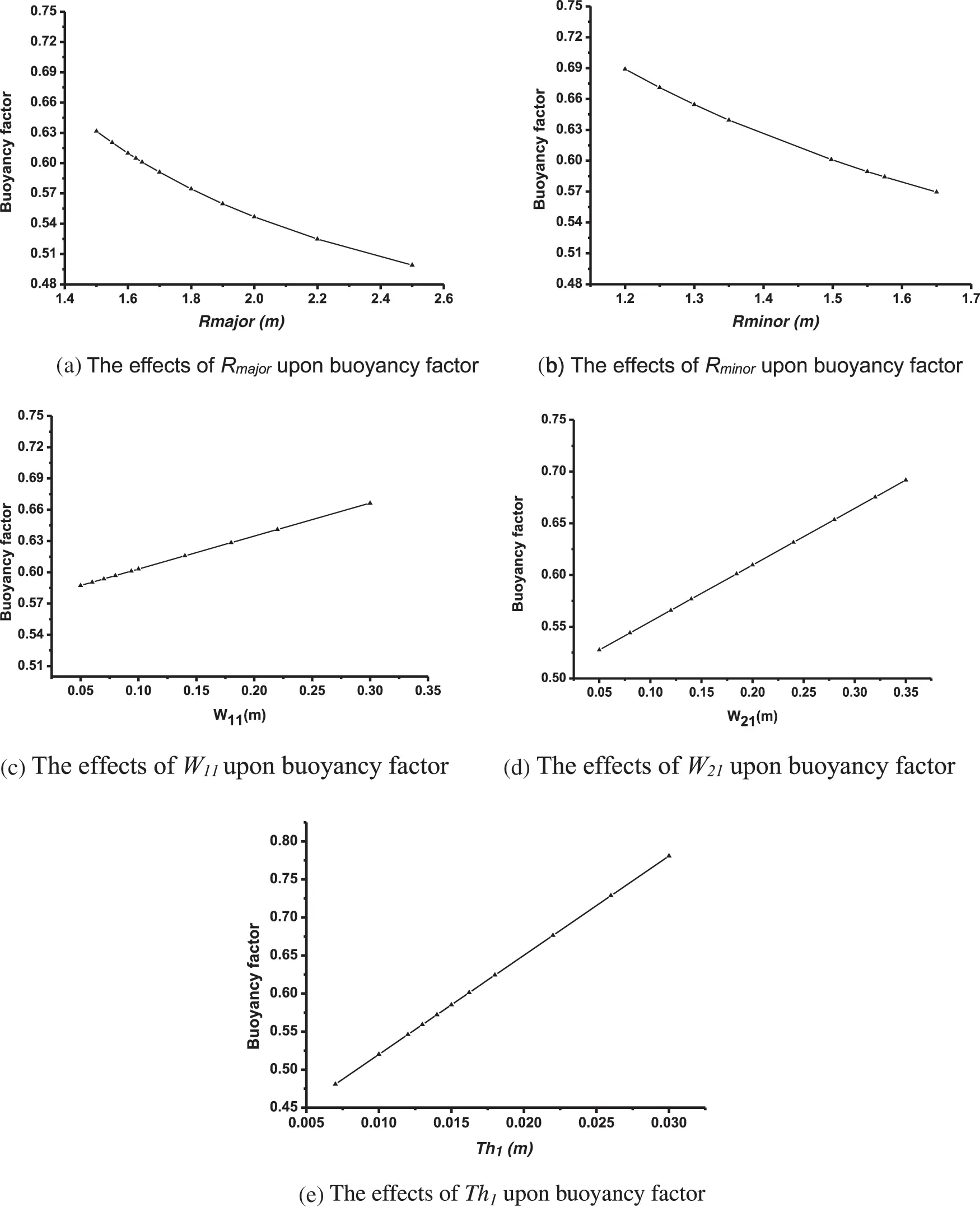Figure 15: The effect of buoyancy factor by design variables

6.2 The Effect of Design Variables on the Deflection Value

The effect of operating depth (H) upon the maximum deflection value is illustrated in Fig. 16a. It is shown that maximum deflection value increases as the operating depth increases. Furthermore, Fig. 16b illustrates that increasing Rmajor can increase the maximum deflection value. Additionally, It is noticed that Rmajor has a higher effect on maximum deflection value compared to H. Additionally; Fig. 16d confirms that maximum deflection value is inversely proportional to shell thickness (Th1), rib width (W11 and W21) and Rminor. The angle (θ) has little effect on the maximum deflection value. Furthermore, the maximum deflection value is nearly constant as (θ) increases.Figure 16: The effect of maximum deflection by design variables

6.3 The Effect of Design Variables on the Buckling Strength Factor

The buckling strength factor is the ratio between the critical buckling loads to operating loading. The pressure-hull is buckle when the actual load exceeds the critical value. To ensure stability, the critical buckling strength must exceed the actual load and must be greater than 1. Fig. 17a shows the effect of operating depth (H) on the buckling strength factor. It is noticed that, the buckling strength factor declines when the operating depth enlarges. Maximum variant take place when H is greater than 2000 m. After that, the buckling strength factor decreases rapidly.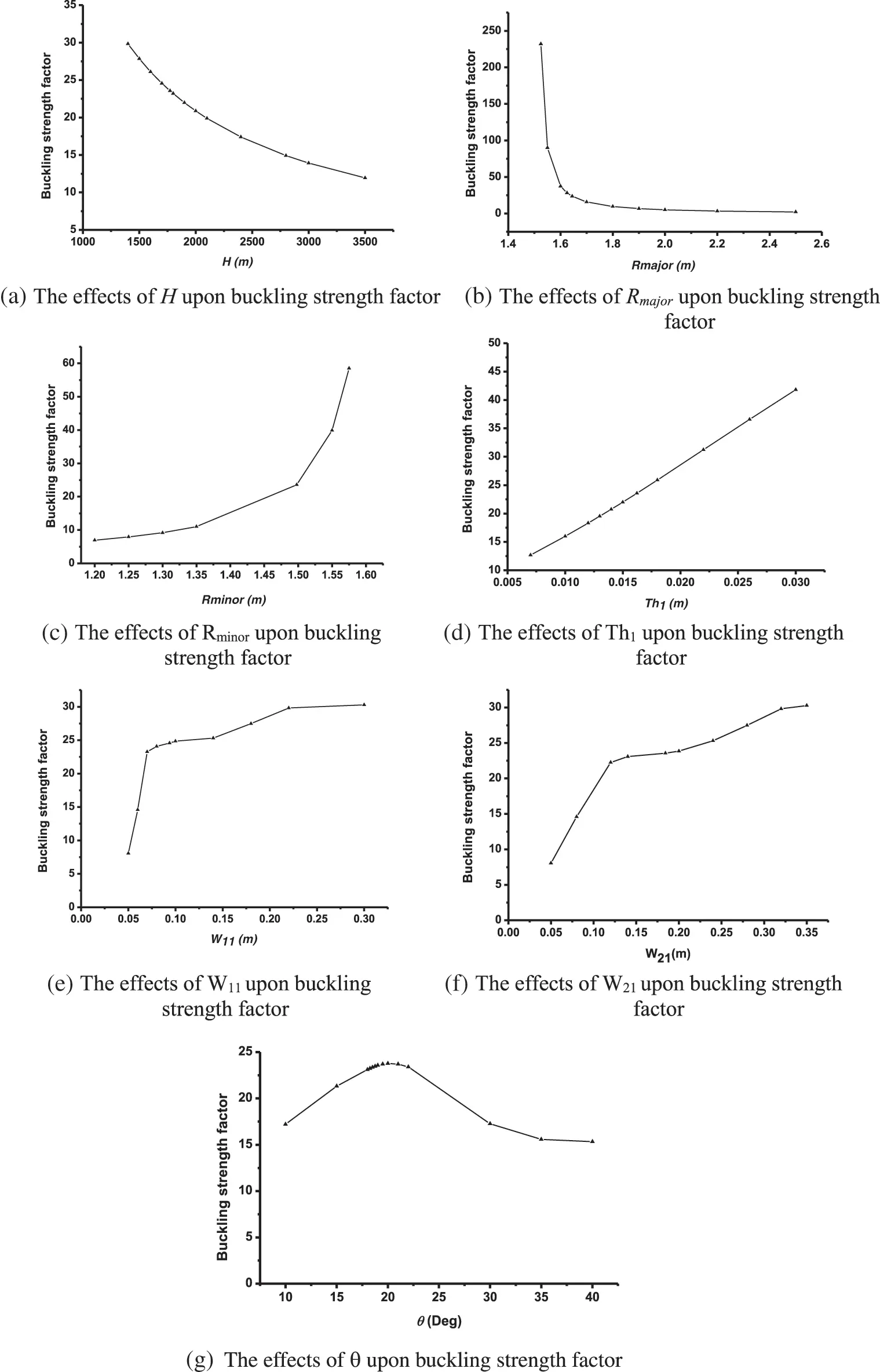Figure 17: The effect of buckling strength factor by design variables

The effect of Rmajor on the buckling strength factor is shown in Fig. 17b. The figure illustrates that increasing Rmajor decreases the buckling strength factor rapidly, and best values occur when Rmajor is ranging from 1.5–1.7 m. After that, as Rmajor increases, the value of the buckling strength factor decreases to the minimum rate. Fig. 17c shows effect of Rminor on the buckling strength factor. The figure illustrates that as Rminor increases, the buckling strength factor increases, and the best performance occurs when Rminor is equal to Rmajor, and the maximum value of the buckling strength factor is achieved to 60. Fig. 17d illustrates the effect of pressure-hull wall thickness (Th1) on the buckling strength factor. The results reveal that, the increase in Th1 increases the buckling strength factor. Figs. 17e, 17f present the effect of W11 and W22 on the buckling strength factor, and illustrate that the two figures have a similar trend. Furthermore, Fig. 17g shows the effect of θ on the buckling strength factor, and indicates that while θ increases, the buckling strength factor increases until θ = 22°. After that, the buckling strength factor decreases rapidly until θ = 30°, and the minimum variation occurs in the range from θ = 30° to 40°.

6.4 The Effect of Design Variables on the Maximum von Mises Stresses

The effect of operating depth (H) on the maximum von Mises stresses is presented in Fig. 18a where the maximum von Mises stress increases as the operating depth increases. Fig. 18b presents the effect of Rmajor on the maximum von Mises stress. As Rmajor increases, maximum von Mises stress is nearly constant. After that, maximum von Mises stress increases linearly as Rmajor increases. Fig. 18c presents the effect of Rminor on maximum von Mises stress value. As Rminor increases, the maximum von Mises stress decreases until Rminor equals 1.49 m. After that, von Mises stresses nearly remain constant. Fig. 18d shows the effect of Th1 on the maximum value of von Mises stress, which is inversely proportional to shell thickness (Th1) until Th1 = 0.016 m. Then, maximum von Mises stress values remain constant as the shell thickness (Th1) increases.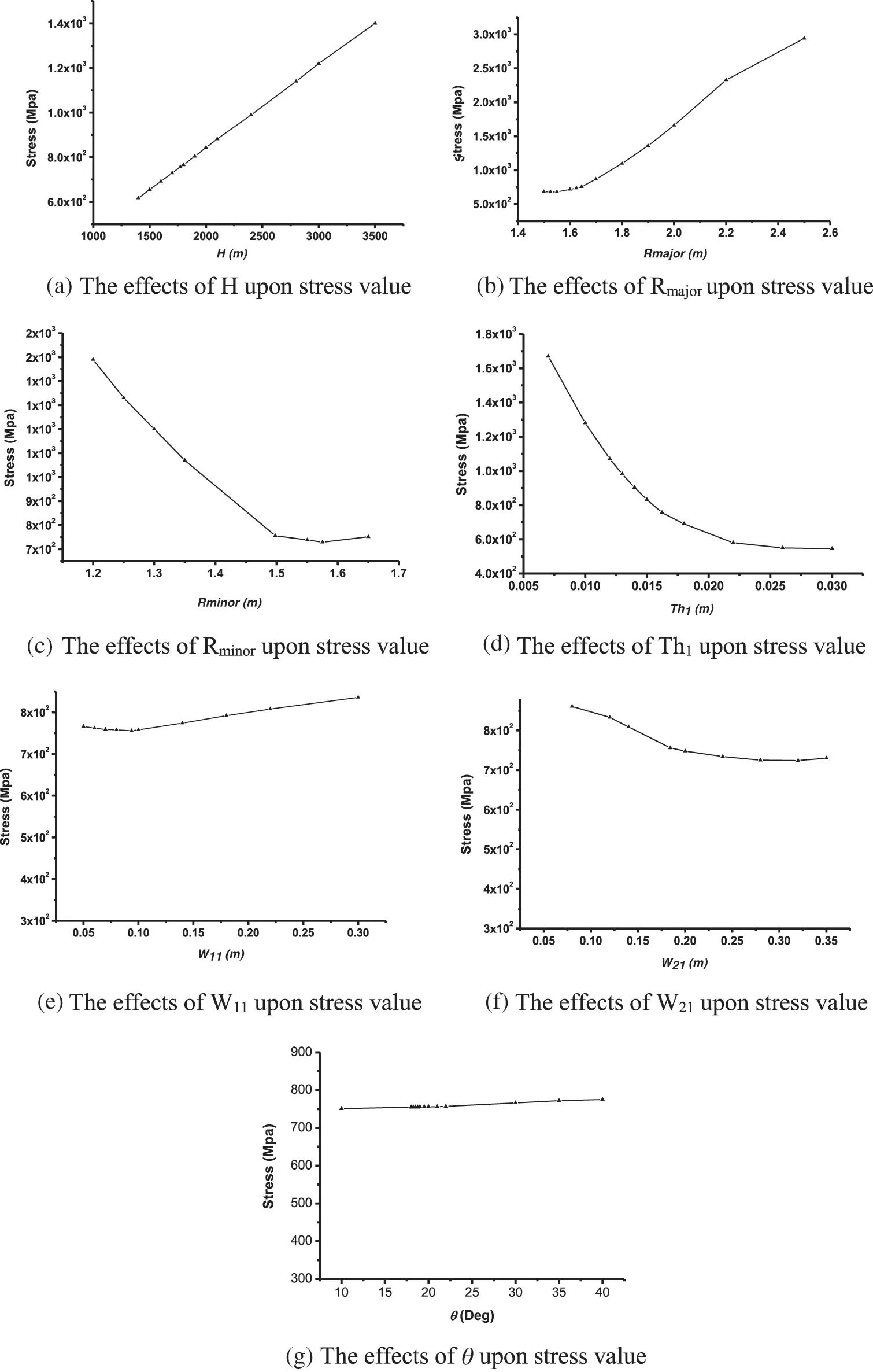Figure 18: The effect of maximum von Mises stress by design variables

Fig. 18e presents the effect of W11 on the value of maximum von Mises stress. The figure reveals that as W11 increases, the maximum von Mises stress decreases until W11 = 0.0937 m. Then the maximum von Mises stress value increases as W11 increases. Fig. 18f demonstrates the effect of W21 on maximum von Mises stress values. The figure reveals that as W21 increases, maximum von Mises stress value decreases, until W21 = 0.3 m. After that, the maximum von Mises stress value increases, as W21 increases. Fig. 18g shows the effect of θ upon the maximum von Mises stress value. The figure indicates that, as θ increases, the maximum von Mises stress values are relatively constant.

7  Conclusions

This paper presents a new model using FEA, optimization and sensitivity analysis on a metallic pressure-hull by improving the design and enhancing the structural capacity by minimizing the B.F. The study illustrated that, the optimal model enhanced the B.F by 31.78% compared to the prototype model. Additionally, B.F was extraordinarily influenced by design variables such as (Rmajor, Rminor and Th1). This model also improved the maximum von Mises stress by 27% compared to the prototype one. Moreover, the design variables (H, Rmajor, Rminor and Th1) have a remarkable influence upon the maximum von Mises stress, however, the design variable (θ) shows a little influence, while the design variables (W11, and W21) have a moderate impact. The maximum deflection value was improved by 57% compared to the prototype model. Moreover, the design variables (H and Rmajor) strongly influence the maximum deflection values, which the deflection increases as these design variables increase. The Buckling strength factor (λ) value decreased with a ratio equal 76% compared to the prototype model. Both material failure and buckling strength factor should be considered in the design of submersible pressure-hull.

Acknowledgement: The authors are grateful to Taif University (Taif, KSA), Mansoura University (Mansoura, Egypt), Military Technical College (Cairo, Egypt) and Future University in Egypt (Cairo, Egypt) for providing all the required facilities to carry out the present research.

Funding Statement: This work was supported by Basic Science Research Program through the National Research Foundation of Korea (NRF) grant funded by the Korea Government (MSIT) (No. NRF-2021R1A2B5B02002599).

Conflicts of Interest: The authors declared no conflicts of interest.

## References

1. B. B. Pan, W. C. Cui, Y. S. Shen and T. Liu, “Further study on the ultimate strength analysis of spherical pressure hulls,” Marine Structures, vol. 23, pp. 444–461, 2010. https://doi.org/10.1016/j.marstruc.2010.1001.
2. F. O. Sonmez, “Optimum design of composite structures: A literature survey (1969–2009),” Journal of Reinforced Plastics and Composites, vol. 36, pp. 3–39, 2016. https://doi.org/10.1177/0731684416668262.
3. D. Pattison, “Design of submarine structures,” SSP74: Defence Procurement Agency Sea Technology Group, Bristol, 2001.
4. C. C. Lian, C. C. Liao and C. M. Lu, “The study of minimum-weight optimal design for submarine pressure hull,” Institute of Technology: Chung Cheng, vol. CCIT-INA-S-MS-Tech.Rept.-78,3. 1989.
5. T. Reynolds, O. Lomacky and M. Krenzke, “Design and analysis of small submersible pressure hulls,” Computers & Structures, vol. 3, pp. 1125–1143, 1973.
6. C. C. Liang, C. Y. Hsuand and H. R. Tsai, “Minimum weight design of submersible pressure hull under hydrostatic pressure,” Computers & Structures, vol. 63, pp. 187–201, 1997. https://doi.org/10.1016/S0045-7949(96)00342-2.
7. D. Mills, Submarine Design and Development, London: Conway Maritime, 1984.
8. F. Elsayed, H. Qi, L. Tong and M. Helal, “Optimal design analysis of composite submersible pressure hull,” in Proceedings of Applied Mechanics and Materials, vol. 578, pp. 89–96, 2014. https://doi.org/10.4028/www.scientific.net/AMM.578-579.89.
9. F. Elsayed, H. Qi, L. Tong and M. Helal, “Design optimization of composite elliptical deep-submersible pressure hull for minimizing the buoyancy factor,” Advances in Mechanical Engineering, vol. 6, pp. 1–15, 2014. https://doi.org/10.1155/2014/987903.
10. M. Helal, H. Huang, D. Wang and E. Fathallah, “Numerical analysis of sandwich composite deep submarine pressure hull considering failure criteria,” Journal of Marine Science and Engineering, vol. 7, pp. 377, 2019. https://doi.org/3390/jmse7100377.
11. M. Helal and E. Fathallah, “Multi-objective optimization of an intersecting elliptical pressure hull as a means of buckling pressure maximizing and weight minimization,” Materials Testing, vol. 61, pp. 1179–1191, 2019. https://doi.org/10.3139/120.111442.
12. K. Abodayeh, A. Raza, M. S. Arif, M. Rafiq, M. Bibi et al., “Numerical analysis of stochastic vector borne plant disease model,” Computers, Materials & Continua, vol. 63, pp. 65–83, 2020. https://doi.org/10.32604/cmc.2020.08838.
13. M. S. Arif, A. Raza, K. Abodayeh, M. Rafiq, M. Bibi et al., “A numerical efficient technique for the solution of susceptible infected recovered epidemic model,” Computer Modeling in Engineering & Sciences, vol. 124, pp. 477–491, 2020. https://doi.org/10.32604/cmes.2020.011121.
14. A. Noor, M. Raza, A., M. S. Arif, M. Rafiq, K. S. Nisar et al., “Non-standard computational analysis of the stochastic covid-19 pandemic model: An application of computational biology,” Alexandria Engineering Journal, vol. 61, pp. 619–630, 2022. https://doi.org/10.1016/j.aej.2021.06.039.
15. A. Raza, A. Ahmadian, M. Rafiq, S. Salahshour and M. Ferrara, “An analysis of a nonlinear susceptible-exposed-infected-quarantine-recovered pandemic model of a novel coronavirus with delay effect,” Results in Physics, vol. 21, pp. 103771, 2021. https://doi.org/10.1016/j.rinp.2020.103771.
16. A. Raza, M. S. Arif, M. Rafiq, M. Bibi, M. Naveed et al., “Numerical treatment for stochastic computer virus model,” Computer Modeling in Engineering & Sciences, vol. 120, pp. 445–465, 2019. https://doi.org/10.32604/cmes.2019.06454.
17. M. Helal and E. Fathallah, “Multi-objective optimization of sandwich composite pressure hull for decreasing weight and drag force and increasing buckling load capacity,” IOP Conference Series: Materials Science and Engineering, vol. 974, pp. 012009, 2020. https://doi.org/10.1088/1757-899x/974/1/012009.
18. B. Li, Y. Pang, X. Zhu and Y. Cheng, “Collaborative optimization and 6σ design for composite pressure hull of underwater vehicle based on lamination parameters,” Journal of Marine Science and Technology, vol. 23, pp. 557–566, 20 https://doi.org/10.1007/s00773-017-0492-4.
19. H. Y. Jung, J. R. Cho, J. Y. Han, W. H. Lee, W. B. Bae et al., “A study on buckling of filament-wound cylindrical shells under hydrostatic external pressure using finite element analysis and buckling formula,” International Journal of Precision Engineering and Manufacturing, vol. 13, pp. 731–737, 2012. https://doi.org/10.1007/s12541-012-0095-2.
20. C. C. Liang, H. W. Chen and C. Y. Jen, “Optimum design of filament-wound multilayer sandwich submersible pressure hulls,” Ocean Engineering, vol. 30, pp. 1941–1967, 2003. https://doi.org/10.1016/S0029-8018(03)00044-1.
21. M. Imran, D. hi, L. Tong, A. Elahi, H. M. Waqas et al., “Multi-objective design optimization of composite submerged cylindrical pressure hull for minimum buoyancy factor and maximum buckling load capacity,” Defence Technology, vol. 17, pp. 1190–1206, 2020. https://doi.org/10.1016/j.dt.2020.06.017.
22. M. Mran, D. Hi, L. Tong, H. M. Waqas, R. Muhammad et al., “Design optimization and non-linear buckling analysis of spherical composite submersible pressure hull,” Materials, vol. 13, pp. 1–20, 2020. https://doi.org/10.3390/ma13112439.
23. M. Helal and E. Fathallah, “Finite element analysis and design optimization of a non-circular sandwich composite deep submarine pressure hull,” Materials Testing, vol. 62, pp. 1025–1032, 2020. https://doi.org/10.3139/120.111580.
24. C. Garland, “Design and fabrication of deep-diving submersible pressure hulls,” SNAME Transactions, vol. 76, pp. 161–179, 1968.
25. G. C. Lee, J. H. Kweon and J. H. Choi, “Optimization of composite sandwich cylinders for underwater vehicle application,” Composite Structures, vol. 96, pp. 691–697, 2013. https://doi.org/10.1016/j.compstruct.2012.08.055.
26. Q. T. Do, V. V. Huynh, S. R. Cho, M. T. Vu, Q. V. Vu et al., “Residual ultimate strength formulations of locally damaged steel stiffened cylinders under combined loads,” Ocean Engineering, vol. 225, pp. 108802, 2021. https://doi.org/10.1016/j.oceaneng.2021.108802.
27. R. Wei, K. Shen and G. Pan, “Optimal design of trapezoid stiffeners of composite cylindrical shells subjected to hydrostatic pressure,” Thin-Walled Structures, vol. 166, pp. 108002, 2021. https://doi.org/10.1016/j.tws.2021.108002.
28. T. Muttaqie, S. H. Park, S. R. Cho and J. M. Sohn, “Optimisation of the design of a steel-welded pressure hull structure based on interactive nonlinear collapse strength analyses,” Ships and Offshore Structures, vol. 17, pp. 1–16, 2020. https://doi.org/10.1080/17445302.2020.1816770.
29. T. Muttaqie, D. Q. Thang, A. R. Prabowo, S. R. Cho and J. M. Sohn, “Numerical studies of the failure modes of ring-stiffened cylinders under hydrostatic pressure,” Structural Engineering and Mechanics, vol. 70, pp. 431–443, 2019. https://doi.org/10.12989/sem.2019.70.4.431.
30. H. C. Viljoen, N. Mahomed, L. H. Cupido and G. P. Mitchell, “Effect of corrosion thinning on depth of operation: Case study of an hy-80 steel submarine pressure hull,” Marine Structures, vol. 81, pp. 103103, 2022. https://doi.org/10.1016/j.marstruc.2021.103103.
31. M. Bagheri, A. A. Jafari and M. Sadeghifar, “Multi-objective optimization of ring stiffened cylindrical shells using a genetic algorithm,” Journal of Sound and Vibration, vol. 330, pp. 374–384, 2011. https://doi.org/10.1016/j.jsv.2010.08.019.
32. M. Walker and R. E. Smith, “A technique for the multiobjective optimisation of laminated composite structures using genetic algorithms and finite element analysis,” Composite Structures, vol. 62, pp. 123–128, 2003. https://doi.org/10.1016/S0263-8223(03)00098-9.
33. L. Costa, L. Fernandes, I. Figueiredo, J. Judice, R. Leal et al., “Multiple and single objective approaches to laminate optimization with genetic algorithms,” Structural and Multidisciplinary Optimization, vol. 27, pp. 55–65, 2004. https://doi.org/10.1007/s00158-003-0355-y.
34. T. Messager, M. Pyrz, B. Gineste and P. Chauchot, “Optimal laminations of thin underwater composite cylindrical vessels,” Composite Structures, vol. 58, pp. 529–537, 2002. https://doi.org/10.1016/S0263-8223(02)00162-9.
35. C. Y. Jen, “Coupled acoustic structural response of optimized ring stiffened hull for scaled down submerged vehicle subject to underwater explosion,” Theoretical and Applied Fracture Mechanics, vol. 52, pp. 96–110, 2009.
36. S. Adali, E. B. Summers and V. E. Verijenko, “Optimisation of laminated cylindrical pressure vessels under strength criterion,” Composite Structures, vol. 25, pp. 305–312, 1993. https://doi.org/10.1016/0263-8223(93)90177-R.
37. C. J. Moon, I. H. Kim, B. H. Choi, J. H. Kweon and J. H. Choi, “Buckling of filament-wound composite cylinders subjected to hydrostatic pressure for underwater vehicle applications,” Composite Structures, vol. 92, pp. 2241–2251, 2010. https://doi.org/10.1016/j.compstruct.2009.08.005.
38. J. Zhang, C. Di, F. Wang and W. Tang, “Buckling of segmented toroids under external pressure,” Ocean Engineering, vol. 239, pp. 109921, 2021. https://doi.org/10.1016/j.oceaneng.2021.109921.
39. K. Y. Maalawi, “Use of material grading for enhanced buckling design of thin-walled composite rings/long cylinders under external pressure,” Composite Structures, vol. 93, pp. 351–359, 2011. https://doi.org/10.1016/j.compstruct.2010.09.007.
40. B. Song, D. Lyu and J. Jiang, “Optimization of composite ring stiffened cylindrical hulls for unmanned underwater vehicles using multi-island genetic algorithm,” Journal of Reinforced Plastics and Composites, vol. 37, no. 10, pp. 668–684, 2018. https://doi.org/10.1177/0731684418760203.
41. J. R. MacKay, M. J. Smith, F. V. Keulen, T. N. Bosman and N. G. Pegg, “Experimental investigation of the strength and stability of submarine pressure hulls with and without artificial corrosion damage,” Marine Structures, vol. 23, pp. 339–359, 2010. https://doi.org/10.1016/j.marstruc.2010.06.001.
42. A. D. Panteleev, “Optimal design of minimum weight sandwich plates and shallow shells,” Applied Mechanics, vol. 20, pp. 103–107, 1984. https://doi.org/10.1007/BF01273674.
43. F. J. J. M. M. Geuskens, O. K. Bergsma., S. Koussios and A. Beukers, “Analysis of conformable pressure vessels:Introducing the multibubble,” AIAA Journal, vol. 49, pp. 10, 2011. https://doi.org/10.2514/1.J050822.
44. Z. Jian, Z. Xinlong, W. Weibo and T. Wenxian, “Overviews of investigation on submersible pressure hulls,” Advances in Natural Science, vol. 7, pp. 1–8, 2014. https://doi.org/10.3968/6129.
45. S. Gesellschaft, Naval Vessels. Hamburg, 2012.
46. R. Craven, D. Graham and J. Dalzel-Job, “Conceptual design of a composite pressure hull,” Ocean Engineering, vol. 128, pp. 153–162, 2016. https://doi.org/10.1016/j.oceaneng.2016.10.031.
47. M. Helal, H. Huang, E. Fathallah, D. Wang, M. M. ElShafey et al., “Numerical analysis and dynamic response of optimized composite cross elliptical pressure hull subject to non-contact underwater blast loading,” Applied Sciences, vol. 9, pp. 3489, 2019. https://doi.org/10.3390/app9173489.
48. C. T. F. Ross, Pressure Vessels External Pressure Technology, Horwood, Chichester, England, British Library, 2011. https://doi.org/10.1533/9780857092496.
49. M. Chen, K. Xie, W. Jia and K. Xu, “Free and forced vibration of ring-stiffened conical–cylindrical shells with arbitrary boundary conditions,” Ocean Engineering, vol. 108, pp. 241–256, 2015, https://doi.org/10.1016/j.oceaneng.2015.07.065.
50. E. Fathallah and M. Helal, “Optimum structural design of deep submarine pressure hull to achieve minimum weight,” The International Conference on Civil and Architecture Engineering, vol. 11, pp. 1–22, 2016. https://doi.org/10.21608/iccae.2016.43445.
51. C. T. F. Ross, “A conceptual design of an underwater missile launcher,” Ocean Engineering, vol. 32, pp. 85–99, 2005. https://doi.org/10.1016/j.oceaneng.2004.04.008.
52. C. S. Smith, “Design of submersible pressure hulls in composite materials,” Marine Structures, vol. 4, pp. 141–182, 1991. https://doi.org/10.1016/0951-8339(91)90018-7.
53. C. Y. Jen and W. H. Lai, “Transient response of multiple intersecting spheres of deep-submerged pressure hull subjected to underwater explosion,” Theoretical and Applied Fracture Mechanics, vol. 48, pp. 112–126, 2007. https://doi.org/10.1016/j.tafmec.2007.05.003.
54. S. Schneider, S. G. Schneider, H. M. Silva and C. d. M. Neto, “Study of the non-linear stress-strain behavior in ti-nb-zr alloys,” Materials Research-Ibero-American Journal of Materials, vol. 8, pp. 435–438, 2005. https://doi.org/10.1590/S1516-14392005000400013.
55. C. C. Liang, S. W. Shiah, C. Y. Jen and H. W. Chen, “Optimum design of multiple intersecting spheres deep-submerged pressure hull,” Ocean Engineering, vol. 31, pp. 177–199, 2004. https://doi.org/10.1016/S0029-8018(03)00120-3.
56. C. T. F. Ross, “A conceptual design of an underwater vehicle,” Ocean Engineering, vol. 33, pp. 2087–2104, 2006. https://doi.org/10.1016/j.oceaneng.2005.11.005.
57. N. Rathinam, B. Prabu and N. Anbazhaghan, “Buckling analysis of ring stiffened thin cylindrical shell under external pressure,” Journal of Ocean Engineering and Science, vol. 6, no. 4, pp. 360–366, 2021. https://doi.org/10.1016/j.joes.2021.03.002.
58. S. I. Wong, “On lightweight design of submarine pressure hulls,” Precision and Microsystems Engineering: Mechanical, Maritime and Materials Engineering, MSc Thesis, pp. 1–181, 2012.
59. J. Tian, C. M. Wang and S. Swaddiwudhipong, “Elastic buckling analysis of ring-stiffened cylindrical shells under general pressure loading via the ritz method,” Thin-Walled Structures, vol. 35, pp. 1–24, 1999. https://doi.org/10.1016/S0263-8231(99)00012-9.
60. J. R. Vinson and R. L. Sierakowski, The Behavior of Structures Composed of Composite Materials, Dordrecht: Springer, 2008. https://doi.org/10.1007/0-306-48414-5.
61. H. Adeli and K. C. Sarma, Cost Optimization of Structures: Fuzzy Logic, Genetic Algorithms, and Parallel Computing, John Wiley & Sons, Shaw, England, 2006.
62. J. Arora, Introduction to Optimum Design, Elsevier, USA, 2011.
63. D. Kalyanmoy, Optimization for Engineering Design: Algorithms and Examples, Phi Learning Pvt. Ltd; New Delhi, India, Feb 1, 2004.
64. E. Fathallah, H. Qi, L. Tong and M. Helal, “Design optimization of lay-up and composite material system to achieve minimum buoyancy factor for composite elliptical submersible pressure hull,” Composite Structures, vol. 121, pp. 16–26, 2015. https://doi.org/10.1016/j.compstruct.2014.11.002.
65. E. Fathallah, H. Qi, L. Tong and M. Helal, “Optimal design analysis of composite submersible pressure hull,” Applied Mechanics and Materials, vol. 578, pp. 89–96, 2014. https://doi.org/10.4028/www.scientific.net/AMM.578-579.89.
66. M. N. Lotfy, E. Fathallah, Y. A. Khalifa and A. K. Dessouki, “Simulation and optimization of a cfrp and a gfrp floating pontoon,” IOP Conference Series: Materials Science and Engineering, vol. 934, pp. 012037, 2020. https://doi.org/10.1088/1757-899x/934/1/012037.
67. A. Alogla, M. Helal, M. M. ElShafey and E. Fathallah, “Numerical analysis for critical structures protection against blast loading using metallic panels,” Applied Sciences, vol. 10, pp. 2121, 2020. https://doi.org/10.3390/app10062121.
68. M. F. M. Fahmy and H. A. Ibrahim, “Steel-frp reinforced concrete moment-resisting frames under lateral loads: Numerical investigation,” Journal of Composites for Construction, vol. 24, pp. 04020064, 2020. https://doi.org/10.1061/(ASCE)CC.1943-5614.0001078.
69. H. A. Ibrahim, M. F. M. Fahmy and Z. Wu, “Numerical study of steel-to-frp reinforcement ratio as a design-tool controlling the lateral response of sfrc beam-column joints,” Engineering Structures, vol. 172, pp. 253–274, 2018. https://doi.org/10.1016/j.engstruct.2018.05.102.
70. M. N. Lotfy, Y. A. Khalifa, A. K. Dessouki and E. Fathallah, “Dynamic behavior of steel and composite ferry subjected to transverse eccentric moving load using finite element analysis,” Applied Sciences, vol. 10, pp. 5367, 2020. https://doi.org/10.3390/app10155367.
71. O. H. Shawki, M. H. Serror and E. Fathallah, “Numerical study on the rotation capacity of sip-strengthened cold-formed steel beams,” Engineering Structures, vol. 251, pp. 1–18, 113542, 2022. https://doi.org/10.1016/j.engstruct.2021.113542.
72. A. Inc, “Ansys theory reference release 14.5,” October edd.; 2012.
73. M. Erdogan, G. Ibrahim, The Finite Element Method and Applications in Engineering Using Ansys, New York: Springer, 2006.
74. S. Ma and H. Mahfuz, “Finite element simulation of composite ship structures with fluid structure interaction,” Ocean Engineering, vol. 52, pp. 52–59, 2012. https://doi.org/10.1016/j.oceaneng.2012.06.010.This work is licensed under a Creative Commons Attribution 4.0 International License, which permits unrestricted use, distribution, and reproduction in any medium, provided the original work is properly cited.